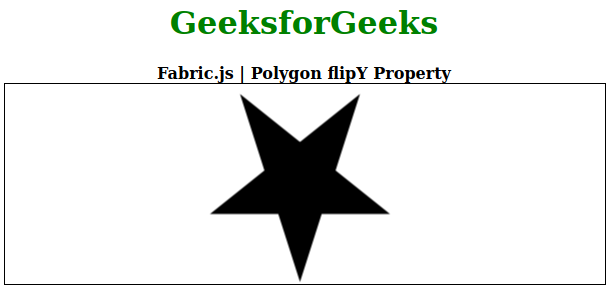Related Articles
Fabric.js Polygon flipY Property
• Last Updated : 26 Aug, 2020

In this article, we are going to see how to set the vertically flip of a canvas polygon in FabricJS. The canvas polygon means the polygon is movable and can be stretched according to requirement. Further, the polygon can be customized when it comes to initial stroke color, height, width, fill color, or stroke width.

Approach: To make it possible we are going to use a JavaScript library called FabricJS. After importing the library, we will create a canvas block in the body tag that will contain the polygon. After this, we will initialize instances of Canvas and polygon provided by FabricJS and use the flipY property to vertically flip the polygon and render the Canvas on the polygon as given in the example below.

Syntax:

```fabric.Polygon([
{ x: pixel, y: pixel },
{ x: pixel, y: pixel },
{ x: pixel, y: pixel},
{ x: pixel, y: pixel},
{ x: pixel, y: pixel }],
{
flipY: boolean
});

```

Parameters: This function accepts a single parameter as mentioned above and described below:

• flipY: It specifies the vertically flip of a canvas object.

Note: Dimension pixels is must create a polygon.

Below examples illustrate the Fabric.JS Polygon flipY property in JavaScript:

Example:

## HTML

 ` ` `<``html``> ` ` `  `<``head``> ` ` `  `    ``` `    ``<``script` `src``= ` `"https://cdnjs.cloudflare.com/ajax/libs/fabric.js/3.6.2/fabric.min.js"``> ` `    `` ` ` ` ` `  `<``body``> ` `    ``<``div` `style``=``"text-align: center;width: 600px;"``> ` `        ``<``h1` `style``=``"color: green;"``> ` `            ``GeeksforGeeks ` `        `` ` `        ``<``b``> ` `            ``Fabric.js | Polygon flipY Property ` `        `` ` `    `` ` `    ``<``canvas` `id``=``"canvas"` `width``=``"600"` `height``=``"200"`  `        ``style``=``"border:1px solid #000000;"``> ` `    `` ` `     `  `    ``<``script``> ` `        ``// Initiate a Canvas instance  ` `        ``var canvas = new fabric.Canvas("canvas"); ` ` `  `        ``// Initiate a polygon instance  ` `        ``var polygon = new fabric.Polygon([ ` `            ``{ x: 295, y: 10 }, ` `            ``{ x: 235, y: 198 }, ` `            ``{ x: 385, y: 78 }, ` `            ``{ x: 205, y: 78 }, ` `            ``{ x: 355, y: 198 }], { ` `            ``flipY: true ` `        ``}); ` ` `  `        ``// Render the polygon in canvas  ` `        ``canvas.add(polygon);  ` `    `` ` ` ` ` `  ` `

Output:My Personal Notes arrow_drop_up
Recommended Articles
Page :